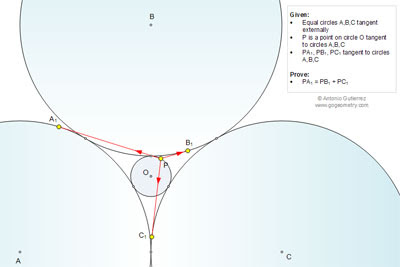## Wednesday, April 23, 2014

### Geometry Problem 1008: Three Equal Tangent Circles, Sum of Tangent Lines, Tangency Points

Level: Mathematics Education, High School, Honors Geometry, College.

Click the figure below to see the complete problem 1008.1.http://s15.postimg.org/hes081d63/pro_1008.png

Let R and r are radii of circles A and O
Let S and T are 2 points on circle O such that PST is an equilateral triangle.
Let d(U, V) denote length of tangent of point U to circle V
Let θ is the angle form by OP and Ax
Note that d(P,B)=d(S,A) ; d(P, B)=d(S, A) and d(P,C)=d(T,A)
The problem become to show that d(P,A)=d(S,A)+d(T,A)…..(1)
With algebra calculation we have d(P,A)= absolute value of ( 2.cos(θ /2). sqrt(r.(R+r)))
d(S,A)= absolute value of ( 2.cos(θ /2+pi/3). sqrt(r.(R+r)))
d(T,A)= absolute value of ( 2.cos(θ /2+2.pi/3). sqrt(r.(R+r)))
Since PST is an equilateral so we always have cos(θ /2)+ cos(θ /2+pi/3)+ cos(θ /2+2.pi/3)=0
And absolute value of 1 term is the summation of absolute values of 2 other terms
and the expression (1) is always true

2.See link below for the sketch
http://s22.postimg.org/e3ndru7w1/pro_1008.png